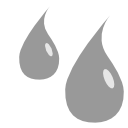# Bloom node¶This documentation is for version 4.0 of Bloom (net.sf.cimg.CImgBloom).

## Description¶

Apply a Bloom filter (Kawase 2004) that sums multiple blur filters of different radii,

resulting in a larger but sharper glare than a simple blur.

It is similar to applying ‘Count’ separate Blur filters to the same input image with sizes ‘Size’, ‘Size’*‘Ratio’, ‘Size’*‘Ratio’^2, etc., and averaging the results.

The blur radii follow a geometric progression (of common ratio 2 in the original implementation, bloomRatio in this implementation), and a total of bloomCount blur kernels are summed up (bloomCount=5 in the original implementation, and the kernels are Gaussian).

The blur filter can be a quasi-Gaussian, a Gaussian, a box, a triangle or a quadratic filter.

Ref.: Masaki Kawase, “Practical Implementation of High Dynamic Range Rendering”, GDC 2004.

Uses the ‘vanvliet’ and ‘deriche’ functions from the CImg library.

CImg is a free, open-source library distributed under the CeCILL-C (close to the GNU LGPL) or CeCILL (compatible with the GNU GPL) licenses. It can be used in commercial applications (see http://cimg.eu).

## Inputs¶

Input Description Optional
Source   No

## Controls¶

Parameter / script name Type Default Function
Size / size Double x: 0 y: 0 Size (diameter) of the filter kernel, in pixel units (>=0). The standard deviation of the corresponding Gaussian is size/2.4. No blur is applied if size < 0.24 (Gaussian and quasi-Gaussian) or <= 1 (box, triangle and quadratic).
Uniform / uniform Boolean Off Apply the same amount of blur on X and Y.
Ratio / bloomRatio Double 2 Ratio between successive kernel sizes of the bloom filter. A ratio of 1 gives no Bloom effect, just the original blur. A higher ratio gives a blur kernel with a heavier tail. The original implementation uses a value of 2.
Count / bloomCount Integer 5 Number of blur kernels of the bloom filter. The original implementation uses a value of 5. Higher values give a wider of heavier tail (the size of the largest blur kernel is 2**bloomCount * size). A count of 1 is just the original blur.
Border Conditions / boundary Choice Nearest
Specifies how pixel values are computed out of the image domain. This mostly affects values at the boundary of the image. If the image represents intensities, Nearest (Neumann) conditions should be used. If the image represents gradients or derivatives, Black (Dirichlet) boundary conditions should be used.
Black (black): Dirichlet boundary condition: pixel values out of the image domain are zero.
Nearest (nearest): Neumann boundary condition: pixel values out of the image domain are those of the closest pixel location in the image domain.
Filter / filter Choice Quasi-Gaussian
Bluring filter. The quasi-Gaussian filter should be appropriate in most cases. The Gaussian filter is more isotropic (its impulse response has rotational symmetry), but slower.
Quasi-Gaussian (quasigaussian): Quasi-Gaussian filter (0-order recursive Deriche filter, faster) - IIR (infinite support / impulsional response).
Gaussian (gaussian): Gaussian filter (Van Vliet recursive Gaussian filter, more isotropic, slower) - IIR (infinite support / impulsional response).
Box (box): Box filter - FIR (finite support / impulsional response).
Triangle (triangle): Triangle/tent filter - FIR (finite support / impulsional response).
Expand RoD / expandRoD Boolean Off Expand the source region of definition by 1.5*size (3.6*sigma).
Crop To Format / cropToFormat Boolean On If the source is inside the format and the effect extends it outside of the format, crop it to avoid unnecessary calculations. To avoid unwanted crops, only the borders that were inside of the format in the source clip will be cropped.
Alpha Threshold / alphaThreshold Double 0 If this value is non-zero, any alpha value below this is set to zero. This is only useful for IIR filters (Gaussian and Quasi-Gaussian), which may produce alpha values very close to zero due to arithmetic precision. Remind that, in theory, a black image with a single white pixel should produce non-zero values everywhere, but a few VFX tricks rely on the fact that alpha should be zero far from the alpha edges (e.g. the premult-blur-unpremult trick to fill holes)). A threshold value of 0.003 is reasonable, and values between 0.001 and 0.01 are usually enough to remove these artifacts.
(Un)premult / premult Boolean Off Divide the image by the alpha channel before processing, and re-multiply it afterwards. Use if the input images are premultiplied.
Invert Mask / maskInvert Boolean Off When checked, the effect is fully applied where the mask is 0.
Mix / mix Double 1 Mix factor between the original and the transformed image.